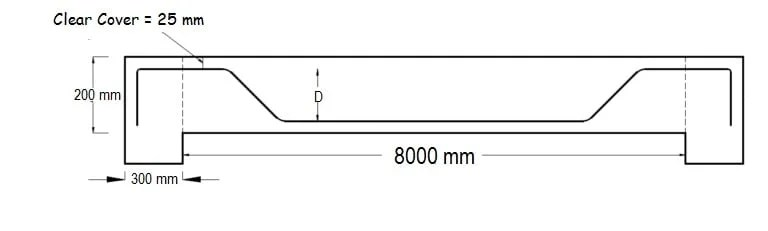## Register Now

Lorem ipsum dolor sit amet, consectetur adipiscing elit.Morbi adipiscing gravdio, sit amet suscipit risus ultrices eu.Fusce viverra neque at purus laoreet consequa.Vivamus vulputate posuere nisl quis consequat.

## Cutting Length Of Bent Up Bars In Slab:

As a site engineer, you need to calculate the cutting length of bars according to the slab dimensions and give instructions to the bar benders.

For small area of construction, you can hand over the reinforcement detailing to the bar benders. They will take care of cutting length. But beware, that must not be accurate. Because they do not give importance to the bends and cranks. They may give some extra inches to the bars for the bends which are totally wrong. So it is always recommended that as a site engineer calculate the cutting length yourself. In this article, we will discuss how to the calculate length for reinforcement bars of slab. Let’s start with an example.

## Example:Where,

Diameter of the bar = 12 mm

Clear Cover = 25 mm

Clear Span (L) = 8000

Slab Thickness = 200 mm

Development Length(Ld) = 40d

## Calculation:Cutting Length = Clear Span of Slab + (2 x Development Length) + (2 x inclined length) – (45° bend x 4) – (90° bend x 2)

Inclined length = D/(sin 45°) – dD/ (tan 45°) = (D/0.7071) – (D/1)= (1D – 0.7071D)/0.7071= 0.42 D

As you can see there are four 45°bends at the inner side (1,2,3 & 4) and two 90° bends ( a,b ).

45° = 1d ; 90° = 2d

Cutting Length = Clear Span of Slab + (2 X Ld) +(2 x 0.42D) – (1d x 4) – (2d x 2) [BBS Shape Codes]

Where,

d = Diameter of the bar.

Ld = Development length of bar.

D = Height of the bend bar.

In the above formula, all values are known except ‘D’.

So we need to find out the value of “D”.

D = Slab Thickness – (2 x clear cover) – (diameter of bar)

= 200 – (2 × 25) – 12

= 138 mm

Now, putting all values in the formula

Cutting Length = Clear Span of Slab + (2 x Ld) +(2 x 0.42D) – (1d x 4) – (2d x 2)

= 8000 + (2 x 40 x 12) +(2 x 0.42 x 138) – (1 x 12 x 4) – (2 x 12 x 2)

Cutting Length = 8980 mm or 8.98 m.

So for the above dimension, you need to cut the main bars 8.98 m in length.